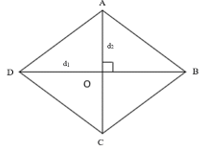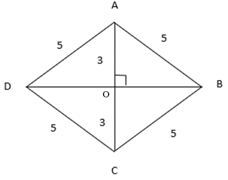# Area of a Rhombus Formula

## Area of a Rhombus Formula - Perimeter and Area of Rhombus

A rhombus is a parallelogram in which adjacent sides are equal (consequently all the sides are equal). The diagonals of a rhombus bisect each other as it is a parallelogram, but they are also perpendicular to each other. We can use this fact to derive a simple and interesting formula for the area of the rhombus. The following figure shows a rhombus whose diagonal lengths are known, say ${d_1}$ and ${d_2}$.We know that the diagonal of a rhombus divides it into two congruent triangles and consequently the two triangles have equal areas. Since the diagonals of a rhombus bisect each other at 90°, we can calculate the height and the base of one of these triangles and multiply the result by two to get the area of the rhombus.
$\begin{gathered} AO = \frac{{AC}}{2} = \frac{{{d_2}}}{2} \hfill \\ {\text{area}}\left( {\Delta ADB} \right) = \frac{1}{2} \times DB \times AO = \frac{1}{2} \times {d_1} \times \frac{{{d_2}}}{2} = \frac{{{d_1}{d_2}}}{4} \hfill \\ {\text{area}}\,{\text{of}}\,{\text{rhombus}} = 2 \times area\left( {\Delta ADB} \right) = 2 \times \frac{{{d_1}{d_2}}}{4} = \frac{{{d_1}{d_2}}}{2} \hfill \\ \end{gathered}$
Let’s look at an example now.

Question:
If the perimeter of a rhombus is 20 cm and one of its diagonals is 6 cm long, calculate its area.
Solution:
${\text{perimeter}} = 4a = 20\,cm \Rightarrow a = \frac{{20}}{4} = 5\,cm$
Since the diagonals of a rhombus bisect each other at 90°, they form four right triangles. Let’s consider one of these right triangles.Since $AC = 6\,cm$, $AO = \frac{{AC}}{2} = 3\,cm$
Since $\Delta AOB$ is a right triangle, we can use the Pythagorean theorem.
$\begin{gathered} A{B^2} = A{O^2} + B{O^2} \Rightarrow BO = \sqrt {A{B^2} - A{O^2}} = \sqrt {25 - 9} = 4\,cm \hfill \\ DB = 2 \times BO = 8\,cm \hfill \\ {\text{area}} = \frac{{{d_1}{d_2}}}{2} = \frac{{6 \times 8}}{2} = 24\,c{m^2} \hfill \\ \end{gathered}$
Why don’t you try to solve the following problem?

Question: One of the diagonals of a rhombus is 3 cm more than the other. If its area is $14\,c{m^2},$ find the lengths of its two diagonals.
Options:
(a) $4\,cm,\,\,7\,cm$
(b) $3\,cm,\,\,6\,cm$
(c) $4.2\,cm,\,\,7.2\,cm$
(d) none of these
${d_1} = x\,cm \Rightarrow {d_2} = \left( {x + 3} \right)\,cm$
$\begin{gathered} {\text{area}} = \frac{{{d_1}{d_2}}}{2} = \frac{{x\left( {x + 3} \right)}}{2} = 14 \Rightarrow {x^2} + 3x - 28 = 0 \hfill \\ {x^2} + 7x - 4x - 28 = 0 \Rightarrow \left( {x + 7} \right)\left( {x - 4} \right) = 0 \Rightarrow x = - 7,\,4 \hfill \\ \end{gathered}$
Since the length of a line segment cannot be negative, ${d_1} = x = 4\,cm \Rightarrow {d_2} = 4 + 3 = 7\,cm$